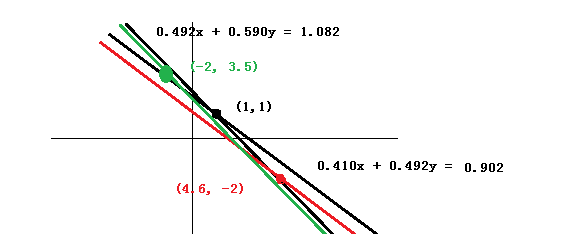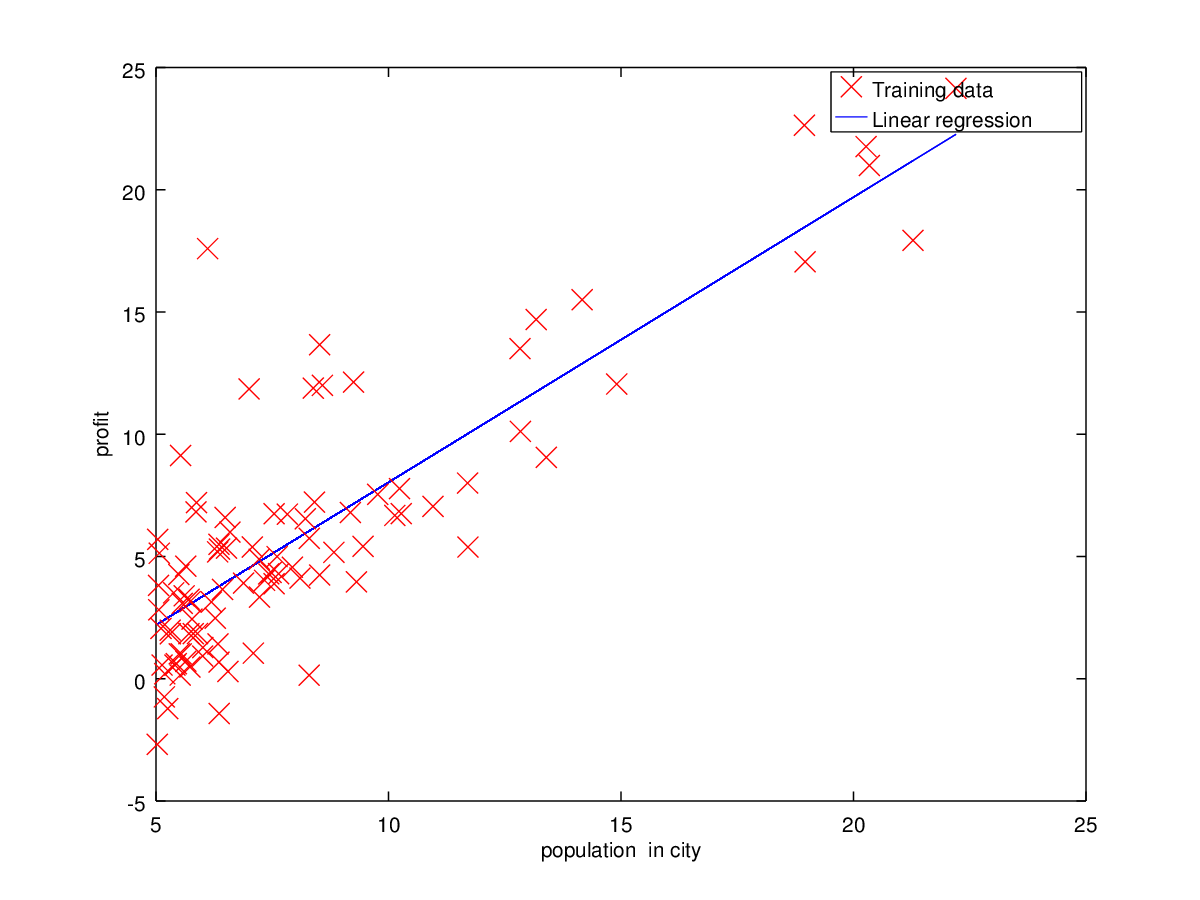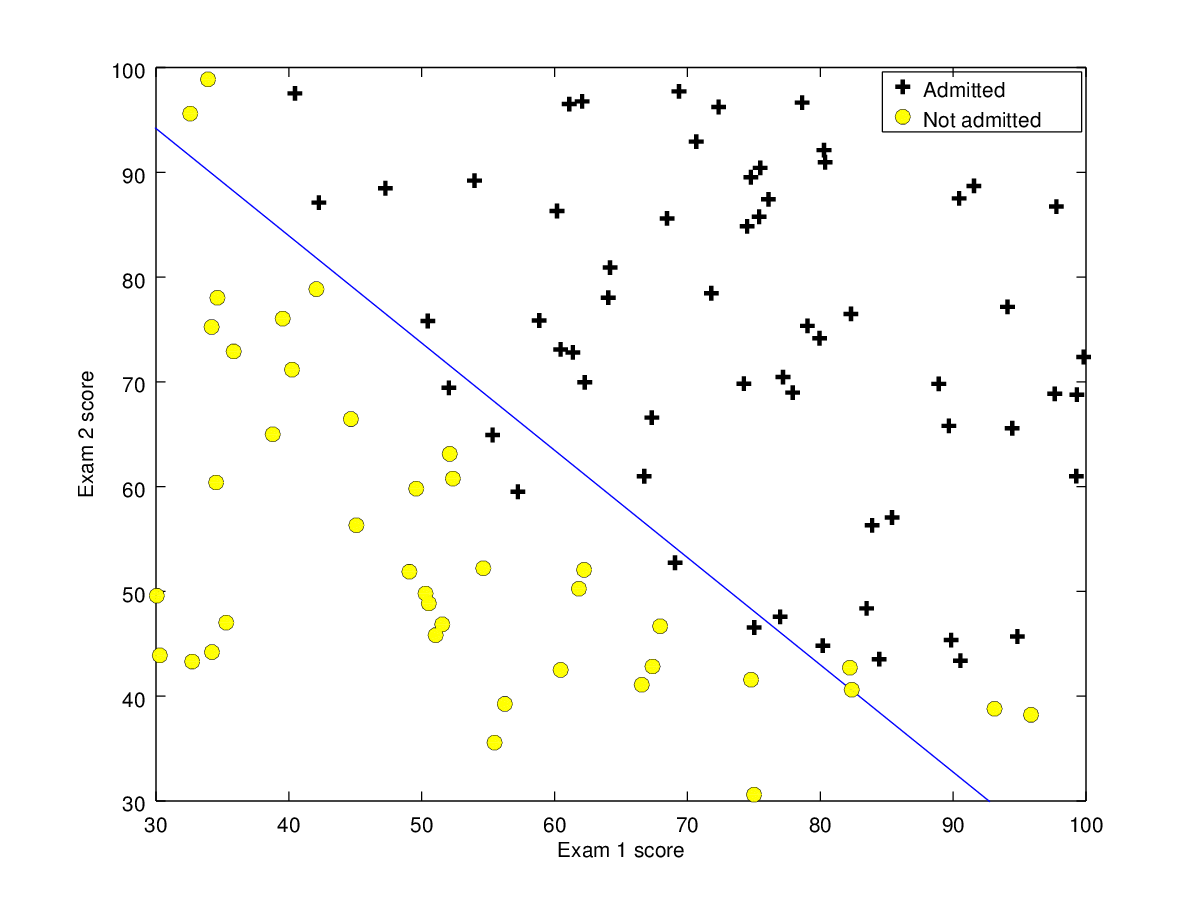# 重修线性代数10——计算精选

10.1 病态系统10.2支点对计算的影响

$A=\begin{bmatrix} 0.0001&1\\ 1&1 \end{bmatrix}=\begin{bmatrix} 1&0\\ 10,000&1 \end{bmatrix}\begin{bmatrix} 0.0001&1\\ 0&-9999 \end{bmatrix}=LU$

$P=\begin{bmatrix} 0&1\\1&0\end{bmatrix},\;PA=\begin{bmatrix} 1&1\\0.0001&1 \end{bmatrix}=\begin{bmatrix}1&0\\0.0001&1\end{bmatrix}\begin{bmatrix} 1&1\\ 0&0.9999 \end{bmatrix}=LU$

10.3 机器学习

n维线性空间中，满足内积〈z,a= b的向量z，构成n维几何空间中的一个n-1维平面，将向量扩充到n+1维空间，令x=(1,zT)Tw=(-b,aT)T，这个内积可以表示为〈x,w= 0.mn维行向量xiT写成m*n矩阵Xmyi列成向量y。设误差函数J(w) =1/2Xw - y^2 = ( Xwy)T ( Xwy)/2，计算问题是，求让J(w)=( Xwy)T ( Xwy)/2取最小值的向量w0.

J(w)是个在n维空间中的二阶幂函数的曲面，它有唯一的极小值在梯度为零处，即梯度X T ( Xwy) =0，这可以表示成正规方程X TXw =X Ty. 它有唯一解w0 =(X TX)-1X Ty.  在大数据的情况下，这个公式解的计算量太大，我们可以采用迭代的方式求解，这通常从任何一个w的初值开始，沿着这个梯度X T ( Xwy)下降的方向，迭代逼近这个极值点w0 .

n维线性空间中，满足内积〈z, a= b的向量x，在a方向上投影的长度都是b/a‖，这些x向量的端点构成的n-1维的平面，这超平面与原点的距离是b/a‖。空间上面的点依指向它的向量za上面的投影被这超平面分成两个部分，依内积〈z, a〉是否大于b，确定它们属于哪一类。这是模式辨识和机器学习中分类最为基本的直观图像。

10.4世界是线性的吗？

10.5 结语

（系列终）

http://blog.sciencenet.cn/blog-826653-1038639.html

## 全部精选博文导读

GMT+8, 2021-10-28 12:37